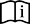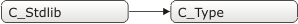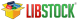ANSI C Stdlib Library

The mikroC PRO for PIC provides a set of standard ANSI C library functions of general utility.Important :
• Not all of the standard functions have been included.
• The functions have been mostly implemented according to the ANSI C standard, but certain functions have been modified in order to facilitate PIC programming. Be sure to skim through the description before using standard C functions.

Library Dependency Treeabs

Prototype int abs(int a); Function returns the absolute (i.e. positive) value of a. result = abs(-12); // result = 12

atof

Prototype double atof(char *s); Function converts the input string s into a double precision value and returns the value. Input string s should conform to the floating point literal format, with an optional whitespace at the beginning. The string will be processed one character at a time, until the function reaches a character which it doesn’t recognize (including a null character). doub = atof("-1.23"); // doub = -1.23

atoi

Prototype int atoi(char *s); Function converts the input string s into an integer value and returns the value. The input string s should consist exclusively of decimal digits, with an optional whitespace and a sign at the beginning. The string will be processed one character at a time, until the function reaches a character which it doesn’t recognize (including a null character). result = atoi("32000"); // result = 32000

atol

Prototype long atol(char *s); Function converts the input string s into a long integer value and returns the value. The input string s should consist exclusively of decimal digits, with an optional whitespace and a sign at the beginning. The string will be processed one character at a time, until the function reaches a character which it doesn’t recognize (including a null character). result = atol("-32560"); // result = -32560

div

Prototype div_t div(int number, int denom); Function computes the result of division of the numerator number by the denominator denom; the function returns a structure of type div_t comprising quotient (quot) and remainder (rem), see Div Structures. dt = div(1234,100);

ldiv

Prototype ldiv_t ldiv(long number, long denom); Function is similar to the div function, except that the arguments and result structure members all have type long. Function computes the result of division of the numerator number by the denominator denom; the function returns a structure of type ldiv_t comprising quotient (quot) and remainder (rem), see Div Structures. dl = ldiv(-123456, 1000);

uldiv

Prototype uldiv_t uldiv(unsigned long number, unsigned long denom); Function is similar to the div function, except that the arguments and result structure members all have type unsigned long. Function computes the result of division of the numerator number by the denominator denom; the function returns a structure of type uldiv_t comprising quotient (quot) and remainder (rem), see Div Structures. dul = uldiv(123456,1000);

labs

Prototype long labs(long x); Function returns the absolute (i.e. positive) value of long integer x. result = labs(-2147483647);

max

Prototype int max(int a, int b); Function returns greater of the two integers, a and b. result = max(123,67); // function returns 123

min

Prototype int min(int a, int b); Function returns lower of the two integers, a and b. result = min(123,67); // function returns 67

rand

Prototype int rand(); Function returns a sequence of pseudo-random numbers between 0 and 32767. The function will always produce the same sequence of numbers unless srand is called to seed the start point. while(1) result = rand() ;

srand

Prototype void srand(unsigned x); Function uses x as a starting point for a new sequence of pseudo-random numbers to be returned by subsequent calls to rand. No values are returned by this function. srand(9);

xtoi

Prototype unsigned xtoi(char *s); Function converts the input string s consisting of hexadecimal digits into an integer value. The input parameter s should consist exclusively of hexadecimal digits, with an optional whitespace and a sign at the beginning. The string will be processed one character at a time, until the function reaches a character which it doesn’t recognize (including a null character). result = xtoi("1FF"); // result = 511

Div Structures

typedef struct divstruct {
int quot;
int rem;
} div_t;

typedef struct ldivstruct {
long quot;
long rem;
} ldiv_t;

typedef struct uldivstruct {
unsigned long quot;
unsigned long rem;
} uldiv_t;
Find them on